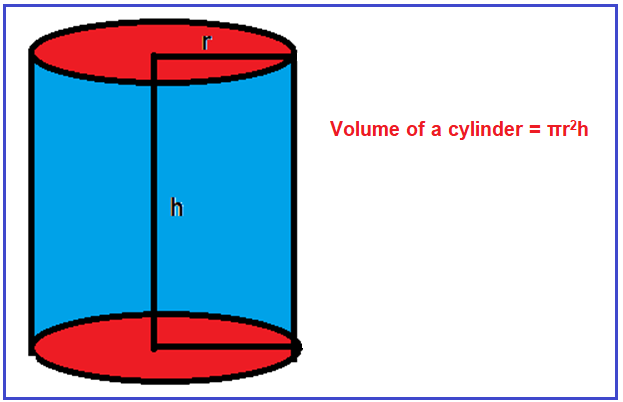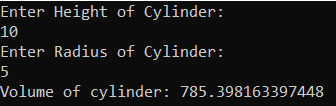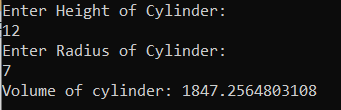# Volume of Cylinder in C#

## The volume of Cylinder Program in C# with Examples

In this article, I am going to discuss how to calculate the Volume of Cylinder in C# with Examples. Please read our previous article, where we discussed How to Calculate the Surface Area of Cylinder in C#. Here, in this article, first, we will discuss what is Cylinder, then we will discuss how to calculate the Volume of the Cylinder and finally, we will see how to implement the Volume of Cylinder program in C#.

##### What is a Cylinder?

A cylinder shape in mathematics is a three-dimensional solid figure which consists of two circular bases connected with two parallel lines. two parallel lines form a curved surface that is bounded by two circles at the top and bottom. The volume of the cylinder can be given by the product of the area of base and height.

Volume of a cylinder = πr2h

where r is the radius of the cylinder and h is the height of the cylinder and Π is the constant value i.e. 3.14 or 22/7. For better understanding, please have a look at the following diagram.Example:
Height (h) = 20 cm
Volume= πr2h
Volume = 3.14 * 142  * 20
Volume = 12315.043202072

##### Logic to Calculate the Volume of Cylinder in C#

To find the Volume of the Cylinder, we need to follow the below steps.

1. We will define the radius, height, pi, and volume variables
2. Then we will assign the value of the radius, height, and pi.
3. then we will calculate the volume of the cylinder by using the formula volume =π*r2*h
4. And the resulting output will store in the volume variable.
##### Example: Volume of Cylinder Program in C#.

The following C# Program will calculate the Volume of the Cylinder.

```using System;
public class VolumeOfCylinder
{
public static void Main()
{
double Height = 20;
Console.WriteLine("Volume of cylinder: " + Volume);
}
}
```

Output: Volume of cylinder: 12315.043202072

##### Example: Taking input from the user

In the following C# program, we will take the cylinder radius and height from the user and then calculate the volume and print it on the console.

```using System;
public class VolumeOfCylinder
{
public static void Main()
{
Console.WriteLine("Enter Height of Cylinder:");

Console.WriteLine("Volume of cylinder: " + Volume);
}
}
```
###### Output:##### C# Code (Using function)

In the below example, we have created one method to calculate the volume of the cylinder.

```using System;
public class VolumeOfCylinder
{
public static void Main()
{
Console.WriteLine("Enter Height of Cylinder:");
Console.WriteLine("Volume of cylinder: " + Volume);